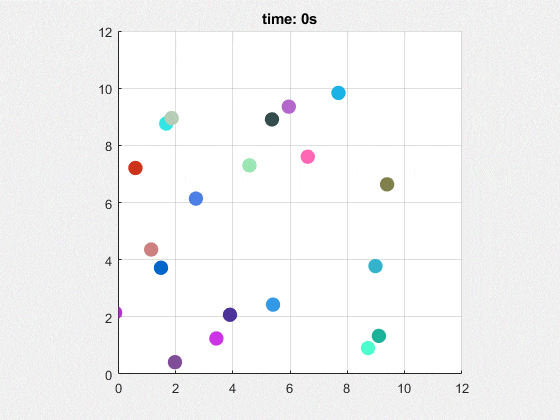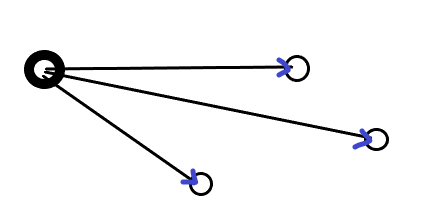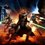# My attempt at an N-body simulation (gravity)

Over the previous year, I learnt about physics simulation and a lot about physics in general, mainly from brilliant. Thanks to those who helped me a lot along the way, Neeraj Anand Badgujar (Talulah Riley), Steven Chase, and Karan Chatrath.First, we need data for each position of the $n$ bodies; you can do this by assigning random initial positions to the $n$ bodies within a certain range.

Build arrays that contain mass data of these bodies, $x$ and $y$ components of the position values, and $x$ and $y$ components of the velocities.

Now, here's how this brute-force CPU simulation works:

First, let's just try to figure out the dynamics of one body, for a small timestep.Forces can be computed by multiplying the unit vectors to each other particle and the magnitudes of the gravitational force.

Essentially, 3 things should be computed each time for the $n-1$ bodies to find the total force exerted onto only 1 of these bodies.

The distance between the single body and each other body, the unit vectors, and finally gravitational force:

$\displaystyle \bold{F} = \frac{Gm_j m_i}{R^2} \hat{u}$

To do this as a program, loop through each of these bodies, and keep a total of the force which you keep updating until you reach the final $n-1$th body.

Then integrate using Euler integration.

This should be done to all the masses. Hence it will result in a nested loop. However, looping from 1 to the $n$th body results in a problem. When you start with the first body, you could be calculating the distance from the first body to the first body, which is zero. Then if you divide by the distance for the unit vector, this would result in division by zero.

So, you should only iterate the algorithm when the indexes of the position data $i$ and $j$ are not equal, ie. so you skip the the $j$th body when doing force computation.

Another consideration: what will happen if both bodies collide perfectly; ie. their centers touch? This would result in division by zero as well in the force computation; we cannot allow that to happen.

In my code I just let each body pass through one and other when they are a certain distance away from each other; this is done by setting the force to zero when they are a small distance away from each other, therefore they just move as per the previous values of their velocity.

However, once they are no longer in that small range, gravitational force again takes precedence.

If you want you can code in some resistive forces, like electrostatic force where all the charges are positive or all are negative, you can just calculate it along with the gravitational force using Coulomb's law. You can also add collision mechanics to it to prevent this error.

Also, when you want to animate each body, it will be a little computationally expensive. I found that using the Matlab rectangle() function to draw a filled circle less expensive than viscircles(). All you have to do is draw the rectangle with curvature and add a random colour to it. I personally added random colour using a colour matrix for random RGB values with row vectors for each body.

Like most of my simulations, I put the dynamics data into arrays which correspond with the time frame of the system and animate separately to the simulation.

However, since there are $n$ bodies, we should have 2 matrices $n \times f$ where $f$ is the number of frames the animation has, one for each component in $x$ and $y$.

The computation does run on the CPU, so wait a few seconds before the animation happens.

  1 2 3 4 5 6 7 8 9 10 11 12 13 14 15 16 17 18 19 20 21 22 23 24 25 26 27 28 29 30 31 32 33 34 35 36 37 38 39 40 41 42 43 44 45 46 47 48 49 50 51 52 53 54 55 56 57 58 59 60 61 62 63 64 65 66 67 68 69 70 71 72 73 74 75 76 77 78 79 80 81 82 83 84 85 86 87 88 89 90 91 92 93 94 95 96 97 98 99 100 101 102 103 104 105 106 107 108 109 110 111 112 113 114 115 116 117 118 119 120 121 122 clc; clear; close all; time = 0; deltaT = 10^-4; G = 1; n = 20; totalForce = [0 0]; times = []; %position data of bodies xPositions = rand(1,n)*(10); yPositions = rand(1,n)*(10); %velocities xVelocities = zeros(n,1); yVelocities = zeros(n,1); %Matrices for animation xPositionMatrix = []; yPositionMatrix = []; %Mass data Masses = []; for i = 1:n Masses = [Masses; 5]; end %% Simulation count = 0; while time <= 10 if mod(count,100) == 0 times = [times; time]; xPositionMatrix = [xPositionMatrix; xPositions]; yPositionMatrix = [yPositionMatrix; yPositions]; end %% dynamics %Outer loop (loop through each body, calculating dynamics of each body %before progressing the simulation for j = 1:n for i = 1:n if i~=j %Distance, unit vector, and total force (which is updated %every iteration currentDistance = hypot(xPositions(i)-xPositions(j),yPositions(i)-yPositions(j)); unitVector = [(xPositions(i)-xPositions(j))/currentDistance (yPositions(i)-yPositions(j))/currentDistance]; if currentDistance <= 0.3 %let bodies pass through each other totalForce = [0 0]; else %calculte gravitational force totalForce = totalForce + unitVector*(G*Masses(j)*Masses(i)/currentDistance^2); end end end %% integrate and update positions for each body %Euler integration for jth body xVelocities(j) = xVelocities(j) + totalForce(1)/Masses(j)*deltaT; yVelocities(j) = yVelocities(j) + totalForce(2)/Masses(j)*deltaT; xPositions(j) = xPositions(j) + xVelocities(j)*deltaT; yPositions(j) = yPositions(j) + yVelocities(j)*deltaT; %remember to reset total force to zero before redoing force %computation for a new jth body! totalForce = [0 0]; end %progress the simulation and recalculate the dynamics for each body time = time + deltaT; count = count + 1; end %% animation axis(gca, "equal"); axis([0 12 0 12]); grid on; particles = []; particleColourMatrix = []; r = 0.5; for i = 1:n %random colour values of the particles particleColourMatrix = [particleColourMatrix; round(rand(1,3),1)]; end for i = 1:length(times) title("time: " + num2str(times(i)) + "s"); for j = 1:n %use rectangle() to draw a filled circle particles = [particles; rectangle('Curvature', [1 1], ... 'Position', [xPositionMatrix(i,j)-r yPositionMatrix(i,j) r r], ... 'facecolor', particleColourMatrix(j,:), 'edgecolor', 'none') ]; end pause(10^-6); if i < length(times) for j = 1:n %delete old elements before redrawing delete(particles(j)); end end %reset the array to zero before refilling the array particles = []; endNote by Krishna Karthik
6 months, 1 week ago

This discussion board is a place to discuss our Daily Challenges and the math and science related to those challenges. Explanations are more than just a solution — they should explain the steps and thinking strategies that you used to obtain the solution. Comments should further the discussion of math and science.

When posting on Brilliant:

• Use the emojis to react to an explanation, whether you're congratulating a job well done , or just really confused .
• Ask specific questions about the challenge or the steps in somebody's explanation. Well-posed questions can add a lot to the discussion, but posting "I don't understand!" doesn't help anyone.
• Try to contribute something new to the discussion, whether it is an extension, generalization or other idea related to the challenge.

MarkdownAppears as
*italics* or _italics_ italics
**bold** or __bold__ bold
- bulleted- list
• bulleted
• list
1. numbered2. list
1. numbered
2. list
Note: you must add a full line of space before and after lists for them to show up correctly
paragraph 1paragraph 2

paragraph 1

paragraph 2

[example link](https://brilliant.org)example link
> This is a quote
This is a quote
    # I indented these lines
# 4 spaces, and now they show
# up as a code block.

print "hello world"
# I indented these lines
# 4 spaces, and now they show
# up as a code block.

print "hello world"
MathAppears as
Remember to wrap math in $$ ... $$ or $ ... $ to ensure proper formatting.
2 \times 3 $2 \times 3$
2^{34} $2^{34}$
a_{i-1} $a_{i-1}$
\frac{2}{3} $\frac{2}{3}$
\sqrt{2} $\sqrt{2}$
\sum_{i=1}^3 $\sum_{i=1}^3$
\sin \theta $\sin \theta$
\boxed{123} $\boxed{123}$

Sort by:

Nice simulation. The singularity problem is a tricky one. Another way to avoid these (at the expense of modifying gravity) would be to use the following expression for the strength of the attractive force between particles.

$F_{attract} = G m_1 m_2 \Big(\frac{1}{r^2} - \frac{r_0}{r^3} \Big)$

Let $r$ be the distance between particles. For $r > r_0$, the force is attractive, and for $r < r_0$ the force is repulsive. So as long as the particles don't have too much kinetic energy, they will repel before they can touch, and then start attracting again as they move apart.

- 6 months ago

Ah, perfect. Yes, this looks like quite a neat trick. Also pretty computationally cheap since I can finally get rid of the extra if-else statement.

- 6 months ago

Also, these orbit problems tend to be badly behaved, numerically. And I suspect that with many particles, they will be very badly behaved. If you run with time steps spanning several orders of magnitude, and monitor the ending position of one of the particles, do you get consistent results for all trials?

- 6 months ago

@Steven Chase I've done some editing now, and after adding the modified equation you gave me, it's starting to look just like the particles are colliding and bouncing off. Looking pretty epic. Where did you get that equation?

By the way, here's the data I'm getting when I set

 1 2 3 4 5 6 7 %position data of bodies xPositions = [0 2 5 6]; yPositions = [0 1 3 4]; %velocities xVelocities = [0 1 2 1]; yVelocities = [0 1 3 4]; 

Here I've displayed the values of xPositions and yPositions for different timestep values.

• 10^-3

$x = [10.6400, 7.1220, 17.6220, 17.6420]$

$y = [9.2190, 8.6939, 35.7979, 34.3727]$

• 10^-4

$x = [10.6526, 7.1167, 17.6081, 17.6252]$

$y = [9.2108, 8.6875, 35.7693, 34.3406]$

• 10^-5

$x = [10.6539, 7.1162, 17.6067, 17.6236]$

$y = [9.2100, 8.6869, 35.7665, 34.3374]$

• 10^-6

$x = [10.6540, 7.1161, 17.6065, 17.6234]$

$y = [9.2099, 8.6869, 35.7662, 34.3371]$

In terms of precision, it appears to be quite badly behaved, but for graphing and plotting it will look roughly the same.

I read somewhere that Euler's method doesn't conserve energy or momentum well when solving orbits. There are methods specialised for second order ODEs that arise in orbits, but they are either too complicated for me or too slow. Either way, it's kind of sad that this code is running solely on the CPU. If I did implement this using CUDA (it would give a raw data output) and animated the plot using Matlab, or OpenGL or something, it would be much much faster. For now, I suppose a 10^-5 timestep gives accurate enough results for 4 bodies.

However, I can only hope the result for 20 bodies isn't too inaccurate. I will post a couple of new gifs with the modified equation, it looks spectacular.

- 6 months ago

Glad to hear it. I look forward to seeing the new graphics. I made the equation based on inspiration from the Lennard Jones intermolecular potential.

https://en.wikipedia.org/wiki/Lennard-Jones_potential

I was also reading about integration schemes for orbital calculations. It seems that there is a class of integrators called "symplectic integrators" that are ideal for these applications. I don't understand how they work

https://en.wikipedia.org/wiki/Symplectic_integrator

Also, I presume that the CUDA speedup is based on parallelism (such as exists in many-body systems). But do you have to specially write your code and tell the CUDA cores how to split the work? If so, it sounds like a daunting task.

- 6 months ago

@Steven Chase The equation's pretty simple and a nice model for repulsive forces.

Yes. Matlab does have the Parallel Computing Toolbox where you can write regular for-loops and Matlab automatically parallelises them for you, but it requires a purchase. However, there is a free trial.

If you want to have total control over the parallelisation, you'd have to code the CUDA kernels in yourself. Which is pretty hard.

Another alternative is OpenGL. You can avoid kernels and low level GPU coding by simulating nbodies inside the shaders, however this forces a graphical output as well. Shaders work in parallel too. By doing computation inside a fragment shader itself, you can produce a graphical output and do simulation quickly.

Since I'm using Matlab, I suppose I better start getting the works by starting out parallel computing with the toolbox.

- 6 months ago